Gauge your potential with Aakash BYJU'S JEE Main'23 Mock Test Gauge your potential with Aakash BYJU'S JEE Main'23 Mock Test

# JEE Main 2021 March 17 – Shift 1 Physics Question Paper with Solutions

We have provided JEE Main 2021 March 17th Shift 1 Physics Question Paper along with the answer keys to help students get familiar with the different types of questions asked in the exam. Practicing the question papers will help students to improve their speed of solving problems. Students will also find step-by-step solutions which are very easy to understand.

### JEE Main 2021 March 17th Shift 1 Physics Question Paper

Section - A

Question 1. The vernier scale used for measurement has a positive zero error of 0.2 mm. If while taking a measurement it was noted that 'o' on the vernier scale lies between 8.5 cm and 8.6 cm, vernier coincidence is 6, then the correct value of the measurement is ……………………. cm. (least count = 0.01 cm)

1. a. 8.36 cm
2. b. 8.56
3. c. 8.58 cm
4. d. 8.54 cm

Solution:

Reading = MSR + VSD × LC – zero error

Vernier scale Reading = 6 × 0.01 =0.06 cm

Final Reading = 8.5 + 0.006 - 0.02 = 8.54 cm

Question 2. For what value of displacement do the kinetic energy and potential energy of a simple harmonic oscillation become equal?

1. a. x = A/2
2. b. x = 0
3. c. x = ±A
4. d. x = ± A/√2

Solution:

KE = PE

(½)k(A2–X2)= 1/2kX2

Here, k = spring constant

A = amplitude

x = displacement

A2–X2 = X2

2X2 = A2

X2 =A2/2

X = ± A/√2

Question 3. An electron of mass m and a photon have the same energy E. The ratio of the wavelength of an electron to that of the photon is : (c being the velocity of light)

1. a.
$$\left ( \frac{E}{2m} \right )^{1/2}$$
2. b.
$$c(2mE)^{1/2}$$
3. c.
$$\frac{1}{c}\left ( \frac{E}{2m} \right )^{1/2}$$
4. d.
$$\frac{1}{c}\left ( \frac{2E}{m} \right )^{1/2}$$

Solution:

For photon,

$$E =\frac{hc}{\lambda _{p}}$$

$$\lambda _{p}=\frac{hc}{E}------(i)$$

For electron,

$$\lambda _{e}=\frac{h}{\sqrt{2mE}}------(ii)$$

$$\frac{\lambda _{e}}{\lambda _{p}}=\frac{\frac{h}{\sqrt{2mE}}}{\frac{hc}{E}} = \sqrt{\frac{E}{2mc^{2}}}=\frac{1}{c}\left ( \frac{E}{2m} \right )^{1/2}$$

Question 4. A car accelerates from rest at a constant rate for some time after which it decelerates at a constant rate to come to rest. If the total time elapsed is t seconds, the total distance travelled is :

1. a.
$$\frac{\alpha \beta }{2(\alpha +\beta )}t^{2}$$
2. b.
$$\frac{\alpha \beta }{4(\alpha +\beta )}t^{2}$$
3. c.
$$\frac{4\alpha \beta }{(\alpha +\beta )}t^{2}$$
4. d.
$$\frac{2\alpha \beta }{(\alpha +\beta )}t^{2}$$

Solution: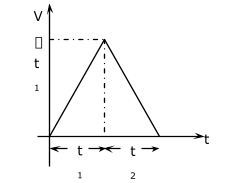Let t be total time travelled

v'→velocity after time t1

then, t1 + t2 = t,

V' = 0 +αt1 (for Ist part of motion)

V = u + at (for IInd part of motion)

0 = α t1 – α t2

t2 = (α/β) t1

t1 +(α/β) t1 = t

$$t_{1}=\frac{\beta }{\alpha +\beta }t$$

Distance = (½) (t1 + t2)× αt1 area of triangle

$$=\frac{1}{2}t\times \frac{\beta }{\alpha +\beta }t$$

$$=\frac{\alpha \beta }{2(\alpha +\beta) }t^{2}$$

Question 5. A Carnot's engine working between 400 K and 800 K has a work output of 1200 J per cycle. The amount of heat energy supplied to the engine from the source in each cycle is :

1. a. 1800 J
2. b. 3200 J
3. c. 2400 J
4. d. 1600 J

Solution:

η =1–(T2/T1)

T1 = source’s temperature

T2 = sink’s temperature

η = efficiency of engine

W = work output

Q1= heat supplied to engine

= 1– (400/800)= 0.5

η = 1/2

W/Q1= η

1200/Q1 = 1/2

Q1 = 2400 J

Question 6. A mass M hangs on a massless rod of length l which rotates at a constant angular frequency. The mass M moves with the steady speed in a circular path of constant radius. Assume that the system is in a steady circular motion with constant angular velocity ω. The angular momentum of M about point A is LA which lies in the positive z-direction and the angular momentum of M about point B is LB. The correct statement for this system is: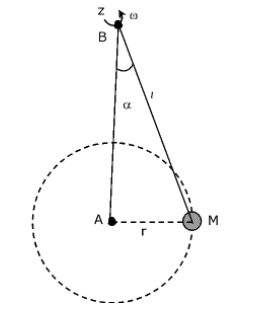1. a. LA and LB are both constant in magnitude and direction
2. b. LB is constant, both in magnitude and direction
3. c. LA is constant, both in magnitude and direction
4. d. LB is constant in direction with varying magnitude

Solution: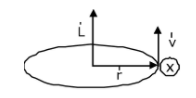Angular momentum,

$$\vec{L}=\vec{r}\times \vec{p}$$
=
$$m(\vec{r}\times \vec{v})$$

The direction of

$$\vec{L}_{A}$$
is along + Z axis and is constant in magnitude (= mvr).But
$$\vec{L}_{B}$$
magnitude is constant and direction is varying with respect to time.

Question 7. Two ideal polyatomic gases at temperatures T1 and T2 are mixed so that there is no loss of energy. If F1 and F2, m1 and m2, n1 and n2 be the degrees of freedom, masses, the number of molecules of the first and second gas respectively, the temperature of the mixture of these two gases is :

1. a.
$$\frac{n_{1}F_{1}T_{1}+n_{2}F_{2}T_{2}}{n_{1}F_{1}+n_{2}F_{2}}$$
2. b.
$$\frac{n_{1}F_{1}T_{1}+n_{2}F_{2}T_{2}}{F_{1}+F_{2}}$$
3. c.
$$\frac{n_{1}F_{1}T_{1}+n_{2}F_{2}T_{2}}{n_{1}+n_{2}}$$
4. d.
$$\frac{n_{1}T_{1}+n_{2}T_{2}}{n_{1}+n_{2}}$$

Solution:

initial internal energy = final internal energy (assuming no internal energy loss)

$$\frac{F_{1}}{2}n_{1}RT_{1}+\frac{F_{2}}{2}n_{}RT_{2}= \frac{F_{1}}{2}n_{1}RT+\frac{F_{2}}{2}n_{}RT$$

$$T = \frac{F_{1}n_{1}T_{1}+F_{2}n_{2}T_{2}}{F_{1}n_{1}+F_{2}n_{2}}$$

Question 8. The output of the given combination gates represents :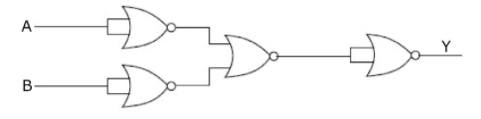1. a. XOR Gate
2. b. NOR Gate
3. c. NAND Gate
4. d. AND Gate

Solution:

Apply De Morgan’s theorem to evaluate output at each gate. We get,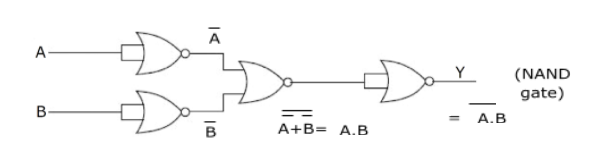Question 9. A triangular plate is as shown. A force is

$$\vec{F}=4\hat{i}-3\hat{j}$$
applied at point P. The torque at point P with respect to point 'O' and 'Q' are :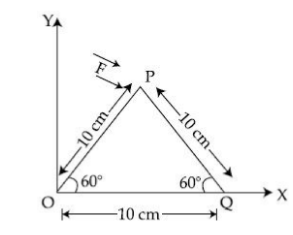1. a. 15 – 20√3, 15 + 20√3
2. b. 15 + 20√3, 15 – 20√3
3. c. –15 + 20√3, 15 + 20√3
4. d. –15–20√3, 15 – 20√3

Solution:

Given:

$$\vec{F}=4\hat{i}-3\hat{j}$$

$$\vec{\tau _{O}}=\vec{r_{1}}\times \vec{F}$$

$$\left ( 5\hat{i}+5\sqrt{3}\hat{j} \right )\times \left ( 4\hat{i}-3\hat{j} \right )$$

$$= - 15\hat{k}-20\sqrt{3}\hat{k}$$

$$\vec{r_{2}}=5\hat{i}+5\sqrt{3}\hat{j}-10\hat{i}$$

$$\vec{r_{2}}=-5\hat{i}+5\sqrt{3}\hat{j}$$

Torque of Q,

$$\vec{\tau _{Q}}=\vec{r_{2}}\times \vec{F}$$

$$\left (- 5\hat{i}+5\sqrt{3}\hat{j} \right )\times \left ( 4\hat{i}-3\hat{j} \right )$$

$$=15\hat{k}-20\sqrt{3}\hat{k}$$

$$=(15-20\sqrt{3})\hat{k}$$

Question 10. A modern grand-prix racing car of mass m is travelling on a flat track in a circular arc of radius R with a speed v.If the coefficient of static friction between the tyres and the track is μs, then the magnitude of negative lift F1acting downwards on the car is : (Assume forces on the four tyres are identical and g = acceleration due to gravity)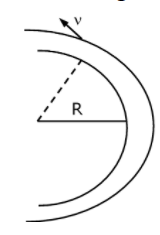1. a.
$$m(\frac{v^{2}}{\mu _{s}R}-g)$$
2. b.
$$m(\frac{v^{2}}{\mu _{s}R}+g)$$
3. c.
$$m(g-\frac{v^{2}}{\mu _{s}R})$$
4. d.
$$- m(g+\frac{v^{2}}{\mu _{s}R})$$

Solution: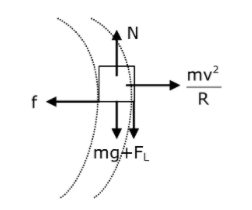Static friction = f =mv2/R from force balance along radial direction,

Normal reaction = N =mv2/Rfrom force balance along vertical,

μ(mg + FL) = mv2/R

mg + FL = mv2/Rμ

FL = (mv2/μR) – mg

= m(v2/μR - g)

Question 11. The thickness at the centre of a plano-convex lens is 3 mm and the diameter is 6 cm. If the speed of light in the material of the lens is 2 × 108 mm-1. The focal length of the lens kept in the air is ____.

1. a. 0.30 cm
2. b. 1.5 cm
3. c. 15 cm
4. d. 30 cm

Solution: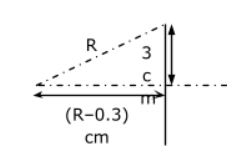R2 = 32 + (R – 0.3)2

R2 = 9 + R2 + 0.09 – 2 × 0.3R

2 × 0.3 R = 9.09

R = 15.15 cm

μ = C/V = 1.5

1/f = (1.5 –1) (1/R)

f= 30 cm

Question 12. If an electron is moving in the nth orbit of the hydrogen atom, then its velocity (vn) for the nth orbit is given as :

1. a. vn ∝ n
2. b. vn ∝ (1/n)
3. c. vn ∝ n2
4. d. vn ∝ 1/n2

Solution:

Velocity of electron of the hydrogen atom in nth orbit is,

$$v_{n}=\frac{e^{2}z}{2n\varepsilon _{0}h}$$

vn∝ 1/n

Therefore, v ∝ 1/n (as z = 1)

Question 13. An AC current is given by I=I1 sinωt + I2 cosωt. A hot wire ammeter will give a reading :

1. a.
$$\frac{I_{1}+I_{2}}{\sqrt{2}}$$
2. b.
$$\sqrt{\frac{I_{1}^{2}+I_{2}^{2}}{2} }$$
3. c.
$$\sqrt{\frac{I_{1}^{2}-I_{2}^{2}}{2} }$$
4. d.
$$\frac{I_{1}+I_{2}}{2\sqrt{2}}$$

Solution:

$$\begin{array}{l} \mathrm{I}_{\mathrm{RMS}}=\sqrt{\frac{\left[I^{2} d t\right.}{\mid d t}} \\ \mathrm{I}_{\mathrm{RMS}}^{2}=\int_{0}^{T} \frac{\left(I_{1} \sin \omega t+I_{2} \cos \omega t\right)^{2} d t} \ \end{array}$$

$$= \frac{1}{T}\int_{0}^{T}(I^{2}_{1}sin^{2}\omega t+I^{2}_{2}cos^{2}\omega t+2I_{1}I_{2}sin\omega tcos\omega t)$$

$$=\frac{I_{1}^{2}}{2}+\frac{I_{2}^{2}}{2}+0$$

$$\mathrm{I}_{\mathrm{RMS}}=\sqrt{\frac{I_{1}^{2}+I_{2}^{2}}{2}}$$

Question 14. Two identical metal wires of thermal conductivities K1 and K2 respectively are connected in series. The effective thermal conductivity of the combination is :

1. a.
$$\frac{2K_{1}K_{2}}{K_{1}+K_{2}}$$
2. b.
$$\frac{K_{1}+K_{2}}{K_{1}K_{2}}$$
3. c.
$$\frac{K_{1}+K_{2}}{2K_{1}K_{2}}$$
4. d.
$$\frac{K_{1}K_{2}}{K_{1}+K_{2}}$$

Solution: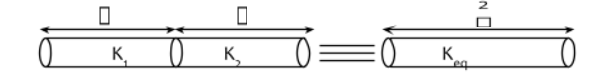Req = R1+R2

$$\frac{1}{K_{eq}}\frac{2l}{A}=\frac{l}{K_{1}A}+\frac{1}{K_{2}A}$$

$$\frac{2}{K_{eq}}=\frac{l}{K_{1}}+\frac{1}{K_{2}}$$

$$\frac{2}{K_{eq}}=\frac{K_{1}+K_{2}}{K_{1}K_{2}}$$

$$K_{eq}=\frac{2K_{1}K_{2}}{K_{1}+K_{2}}$$

Question 15. A boy releases a 0.5 kg ball on the frictionless floor with the speed of 20 ms-1. The ball gets deflected by an obstacle on the way. After deflection it moves with 5% of its initial kinetic energy. What is the speed of the ball now ?

1. a. 14.41 ms–1
2. b. 1.00 ms–1
3. c. 19.0 ms–1
4. d. 4.47 ms–1

Solution:

K.E.f = 5% KEi (since it is a frictionless floor, rolling will not take place)

(½) mv2 = (5/100) × (½) × m ×202

v2 = 1/20× 202 = 20

v = √20 = 2√5 m/s

= 4.47 m/s

Question 16. A polyatomic ideal gas has 24 vibrational modes. What is the value of γ?

1. a. 1.03
2. b. 1.30
3. c. 10.3
4. d. 1.37

Solution:

f = 3T + 3R + 24×2V

= 54

γ = 1+ (2/f)

f = number of degrees of freedom due to translation, rotation, vibration

γ = 1+ (2/54)

=1+ 1/27 =28/27

= 1.03

Question 17. A current of 10 A exists in a wire of cross-sectional area of 5 mm2 with a drift velocity of 2 × 10-3 ms-1. The number of free electrons in each cubic meter of the wire is ________

1. a. 1 × 1023
2. b. 2 × 106/
3. c. 2 × 1025
4. d. 625 × 1025

Solution:

Current through a wire is given by,

I = neAVd

n =I/eAVd(n→ free electron density)

$$=\frac{10}{1.6\times 10^{-19}\times 5\times 10^{-6}\times 2\times 10^{-3}}$$

=6.25×1027= 625 × 1025

Question 18. A solenoid of 1000 turns per metre has a core with a relative permeability of 500. Insulated windings of the solenoid carry an electric current of 5 A. The magnetic flux density produced by the solenoid is: (Permeability of free space = 4 × 10-7 H/m)

1. a. 2 × 10-3 πT
2. b. π/5
3. c. 10-4πT
4. d. πT

Solution:

Magnetic flux density (B) will be,

B = μn i

B = μrμ0 ni

B = 500 × 4π × 10-7 × 103 × 5

B = π × 10-3 × 103

B = π T

Question 19. When two soap bubbles of radii a and b (b > a) coalesce, the radius of curvature of common surface is -

1. a. (b-a/ab)
2. b. ab/(b-a)
3. c. ab/(a+b)
4. d. (a+b)/ab

Solution: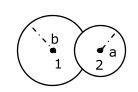P1– P0 = 4S/b

P2– P0 = 4S/a

P2– P1 = 4S/R→excess pressure at common surface

equation (2)–equation(1) = equation(3)

(1/a) – (1/b) =1/R

Therefore, R = ab/(b-a)

Question 20. Which level of the single ionized carbon has the same energy as the ground state energy of hydrogen atom?

1. a. 8
2. b. 1
3. c. 6
4. d. 4

Solution:

En = –13.6(Z2/n2)(energy in nth state for hydrogen-like atom)

Enth of Carbon = E1st of Hydrogen

–13.6× (62/n2) = –13.6 × (12/12)

n = 6

Section – B

Question 21. A parallel plate capacitor whose capacitance C is 14pF is charged by a battery to a potential difference V = 12 V between its plates. The charging battery is now disconnected and a porcelin plate with k = 7 is inserted between the plates, then the plate would oscillate back and forth between the plates, with a constant mechanical energy of ____ pJ. (Assume no friction)

Solution:

Initial energy stored in capacitor is,

Ui =(½) cv2

= (½) × 14 × (12)2 pJ

= 1008 pJ

Final energy stored in capacitor is,

Uf = Q2/2kC

$$= \frac{(14\times 12)^{2}}{2\times 7\times 14}$$

= 144 pJ

oscillating energy = Ui – Uf

= 1008 – 144

= 864 pJ

Question 22. If 2.5 × 10-6 N average force is exerted by a light wave on a non-reflecting surface of 30 cm2 area during 40 minutes of time span, the energy flux of light just before it falls on the surface is _______ W/cm2. (Round off to the nearest integer) (Assume complete absorption and normal incidence conditions are there)

Solution:

Radiation pressure =Intensity/C (for absorbing surface)

I = P × C

$$I= \frac{2.5 \times 10^{-6}}{30cm^{2}}N \times 3 \times 10^{8} m/s$$

I = 25 W/cm2

Question 23. The following bodies

a. a ring

b. a disc

c. a solid cylinder

d. a solid sphere

of same mass 'm' and radius 'R' are allowed to roll down without slipping simultaneously from the top of the inclined plane. The body which will reach first at the bottom of the inclined plane is________.

[Mark the body as per their respective numbering given in the question]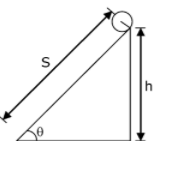Solution:

Acceleration of a body rolling down an inclined plane is,

$$a = \frac{gsin\theta }{(1+\frac{I}{mR^{2}})}$$

IR = mR2, aR = gsinθ/2

ID =mR2/2, aD = (⅔) g sin θ

ISC = mR2/2, asc = (⅔) g sin θ

ISS =(⅖)mR2, ass = (5/7) g sinθ

S = ut +(½) at2,

t = √2S/a

Therefore, t ∝ 1/√a For least time, a should be the highest

⇒ solid spheres will take minimum time.

Question 24. For VHF signal broadcasting,_______ km2 of the maximum service area will be covered by an antenna tower of height 30 m, if the receiving antenna is placed on the ground. Let the radius of the earth be 6400 km. (Round off to the nearest integer) (Take 𝜋 as 3.14)

Solution:

r = √2hR

Area = πr2(consider ‘r’ to be the maximum line of sight distance)

Area = π(2hR) = 3.14×2×30×6400×103 m2

= 1205.76 km2

≈ 1206 km2

Question 25. Consider two identical springs each of spring constant k and negligible mass compared to the mass M as shown. Fig.1 shows one of them and Fig. 2 shows their series combination. The ratio of the time period of oscillation of the two SHM is Tb/Ta = √x, where the value of x is ______. (Round off to the nearest integer)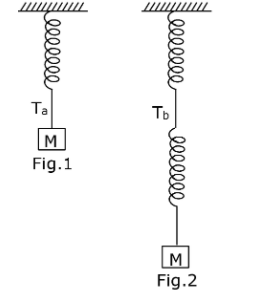Solution:

$$T_{a}= 2\pi \sqrt{\frac{M}{k}}$$

$$k_{eqseries}=\frac{k_{1}k_{2}}{k_{1}+k_{2}}=\frac{k}{2}$$

$$T_{b}= 2\pi \sqrt{\frac{M}{k/2}} = 2\pi \sqrt{\frac{2M}{k}}$$

$$T_{b}= \sqrt{2}T_{a}$$

$$\frac{T_{b}}{T_{a}}= \sqrt{2}$$

Therefore, x = 2

Question 26. Two blocks (m = 0.5 kg and M = 4.5 kg) are arranged on a horizontal frictionless table as shown in figure. The coefficient of static friction between the two blocks is 3/7. Then the maximum horizontal force that can be applied on the larger block so that the blocks move together is _______ N. (Round off to the nearest integer) [Take g as 9.8 ms-2]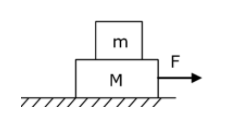Solution:

Maximum acceleration, amax of m (i.e. 0.5 kg) for both blocks to move together

= μg

= (3/7)× 9.8

= 4.2 m/s2

Now, considering both blocks moving together with 4.2 m/s2

Fmax = (M + m) amax

= 4.2×5 = 21 N

Question 27. The radius in kilometre to which the present radius of the earth (R = 6400 km) to be compressed so that the escape velocity is increased 10 times is ______.

Solution:

$$V_{es}=\sqrt{\frac{2GM}{R}}$$

Ves√R= constant

Ves√R = 10Ves√R'

R’ = R/100 = 64 KM

Question 28. The equivalent resistance of a series combination of two resistors is 's'. When they are connected in parallel, the equivalent resistance is 'p'. If s = np, then the maximum value for n is ______. (Round off to the nearest integer)

Solution:

s = np

$$R_{1}+R_{2}=n\left [ \frac{R_{1}R_{2}}{R_{1}+R_{2}} \right ]$$

R12+ R22+ 2R1R2 = nR1R2

R12+(2–n)R1R2 +R12 = 0

(Considering this quadratic equation, for real roots)

b2 – 4ac≥0

[(2–n)R2]2 – 4×1×R22 = 0

(2–n)2R22 = 4R22

2-n = ±2

So n = 4

Question 29. The angular speed of the truck wheel is increased from 900 rpm to 2460 rpm in 26 seconds. The number of revolutions by the truck wheel during this time is ______.

(Assuming the acceleration to be uniform).

Solution:

$$\omega _{f}=2460\times \frac{2\pi }{60}$$

$$\omega _{i}=\frac{900\times2\pi }{60}= 30\pi rad/second$$

$$\alpha =\frac{\omega _{f}-\omega _{i}}{t}$$

$$\alpha =\frac{82\pi -30\pi }{26}$$

$$\theta =\frac{\omega^{2} _{f}-\omega^{2} _{i}}{2\alpha }$$

$$\theta =\frac{(82\pi +30\pi)(82\pi -30\pi ) }{2\times 2\pi }$$

$$\theta =\frac{(112\times 52)\pi ^{2} }{4\pi }$$

θ/2π = no. of revolution

$$\frac{(112\times 52)\pi ^{2} }{4\pi\times 2\pi }$$
= 728

Question 30. Four identical rectangular plates with length, l =2 cm and breadth, b = 3/2 cm are arranged as shown in figure. The equivalent capacitance between A and C is x∈0/d. The value of x is ______. (Round off to the nearest integer)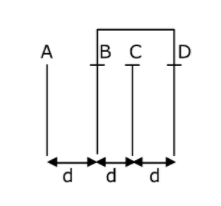Solution: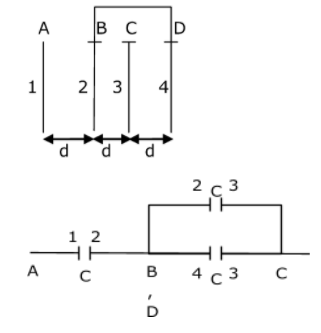$$C=\frac{\epsilon _{0}A}{d}$$

$$C_{eq}=\frac{2C\times C}{2C+C} = \frac{2C}{3}=\frac{2}{3}\frac{\epsilon _{0}A}{d}=\frac{2}{3}\frac{\epsilon _{0}}{d}\times (2\times \frac{3}{2})=\frac{2\epsilon _{0}}{d}$$

### JEE Main 2021 Physics Paper March 17 Shift 1